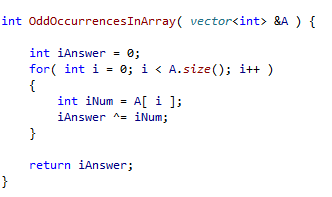You are given an array of N integers where N= {1<= N <= 100,000} and each integer i has value {1<=i<=100,000,000}. One of the integers occurs an odd number of times, whereas all other integers are paired. You are to find the integer that occurs the odd number of times.

Solution

One soultion is to exploit the properties of the bitwise exclusive or operator (^). This operator has the following properties:

• x ^ x = 0
• x ^ 0 = x
• x ^ ~0= ~x (where ~ is the complement)
• x ^ y = y ^ x. XOR is commutative
• (x ^ y ) ^ z = x ^ (y ^ z) . XOR is associative

Therefore from the first two rules, taking the XOR of a number x with itself an even number of times will return 0, and then taking the XOR of zero with x will return x, finding the odd occuring number.

For more uses of XOR, refer to my page on swapping variables